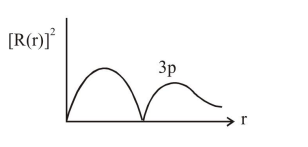# The correct statement about probability density (except at infinite distance from nucleus) is :

Question:

The correct statement about probability density (except at infinite distance from nucleus) is :

1. It $\mathrm{cn}$ be negative for $2 \mathrm{p}$ orbital

2. It can be zero for $3 \mathrm{p}$ orbital

3. It can be zero for $1 \mathrm{~s}$ orbital

4. It can never be zero for $2 \mathrm{~s}$ orbital

Correct Option: , 2

Solution: